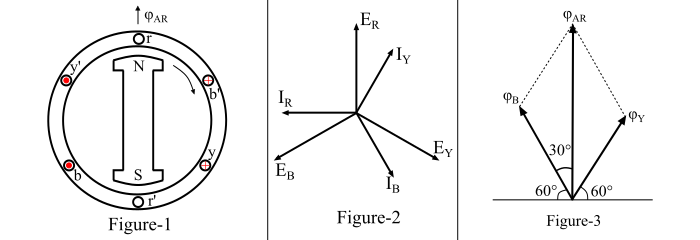Consider a 2-pole alternator as shown in Figure-1. Suppose that the alternator is loaded with an inductive load of zero power factor leading.. Thus, the phase currents 𝐼𝑅 , 𝐼𝑌 and 𝐼𝐵 will be leading their respective phase voltages 𝐸𝑅 , 𝐸𝑌 and 𝐸𝐵 by 90°. Figure-2 shows the phase diagram of the alternator.Now, at time t = 0, the instantaneous values of currents and fluxes are given by,

$$\mathrm{𝑖_{𝑅} = 0;\:\:φ_{𝑅} = 0}$$

$$\mathrm{𝑖_{𝑌} = 𝐼_{𝑚}\:cos 30° = \frac{\sqrt{3}}{2}𝐼_{𝑚};\:\:\:φ_{𝑌} = \frac{\sqrt{3}}{2}φ_{𝑚}}$$

$$\mathrm{𝑖_{𝐵} = −𝐼_{𝑚}\:cos 30° = −\frac{\sqrt{3}}{2}𝐼_{𝑚};\:\:\:φ_{B} = −\frac{\sqrt{3}}{2}φ_{𝑚}}$$

The space diagram of the magnetic fluxes is shown in Figure-3. By the parallelogram law, the resultant armature reaction flux is given by,

$$\mathrm{𝜑_{𝐴𝑅} =\sqrt{φ^{2}_{𝑌} + φ^{2}_{B} + 2 φ_{𝑌}\:φ_{𝐵}\:cos\:60°}}$$

$$\mathrm{\Rightarrow\:φ_{𝐴𝑅} =\sqrt{(\frac{\sqrt{3}}{2}φ_{𝑚})^{2}+(\frac{\sqrt{3}}{2}φ_{𝑚})^{2}+2 \times (\frac{\sqrt{3}}{2}φ_{𝑚})\times (\frac{\sqrt{3}}{2}φ_{𝑚}) \times (\frac{1}{2})}}$$

$$\mathrm{∴\:𝜑_{𝐴𝑅} = 1.5\:φ_{𝑚}}$$

Here, it can be seen that the direction of the armature reaction flux is in the direction of the main field flux. Hence, it will aid the main field flux. It is said to be magnetising flux.

Again, for the successive positions of the rotor, it can be seen that the armature reaction flux φ𝐴𝑅 remains constant in magnitude equal to 1.5 𝜑𝑚 and rotates at synchronous speed. Also, the EMFs induced in each phase of the alternator by the armature reaction flux lag the respective phase currents by 90°.

Therefore, when the alternator supplies a load at leading power factor, then the armature reaction is partly magnetising and partly cross-magnetising. .